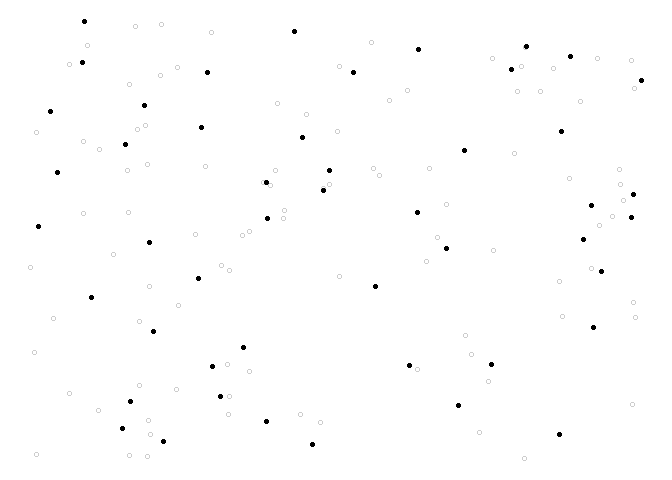# Weakly Associated Vectors (WAVE) Sampling

Spatial data are generally auto-correlated, meaning that if two units selected are close to each other, then it is likely that they share the same properties. For this reason, when sampling in the population it is often needed that the sample is well spread over space. A new method to draw a sample from a population with spatial coordinates is proposed. This method is called `wave` (weakly associated vectors) sampling. It uses the less correlated vector to a spatial weights matrix to update the inclusion probabilities vector into a sample. For more details see Raphaël Jauslin and Yves Tillé (2019) https://arxiv.org/abs/1910.13152.

## Installation

### CRAN version

``install.packages("WaveSampling")``

You can install the latest version of the package `WaveSampling` with the following command:

``````# install.packages("devtools")
devtools::install_github("Rjauslin/WaveSampling")``````

## Simple example

This basic example shows you how to solve a common problem. Spatial coordinates from the function `runif()` are firstly generated.

``````library(WaveSampling)
#> Le chargement a nécessité le package : Matrix

N <- 144
n <- 48
X <- cbind(runif(N),runif(N))
#>            [,1]      [,2]
#>  [1,] 0.4357956 0.3757532
#>  [2,] 0.3778253 0.9184581
#>  [3,] 0.4171640 0.7267468
#>  [4,] 0.4993113 0.4654331
#>  [5,] 0.8498832 0.8845685
#>  [6,] 0.5836470 0.6395404
#>  [7,] 0.6874862 0.3310646
#>  [8,] 0.1087650 0.9061414
#>  [9,] 0.3874497 0.4504327
#> [10,] 0.4484322 0.6564196``````

Inclusion probabilities `pik` is set up all equal with the function `rep()`.

``pik <- rep(n/N,times = N)``

It only remains to use the function `wave()`,

``s <- wave(X,pik)``

We can also generate a plot to observe the result.

``````library(ggplot2)
ggplot() +
geom_point(data = data.frame(x = X[,1],y = X[,2]),
aes(x = x,y = y),
shape = 1,
alpha = 0.2)+
geom_point(data = data.frame(x = X[s == 1,1],y = X[s == 1,2]),
aes(x,y),
shape = 16,
colour = "black")+
theme_void()``````## Performance

As explained on the website of Microsoft R open https://mran.microsoft.com/open, R is designed to use only one thread but could be linked with a multi-threaded version of BLAS/LAPACK. The package is implemented with the package RcppArmadillo http://arma.sourceforge.net/ that provide an integration of various matrix decompositions with LAPACK library. Intel MKL that is used by the Microsoft R open use a multi-threaded version of BLAS/LAPACK. Hence the package could gain time from the Microsoft R open. (Not tested, but linking R with OpenBLAS should also work).

``````N <- 500
n <- 100
X <- as.matrix(cbind(runif(N),runif(N)))
pik <- rep(n/N,N)
system.time(s <- wave(X,pik,tore = T,shift =T,comment = T))``````

#### R 3.6.1

``````user        system    elapsed
42.96       0.67      43.74``````

#### MRO 3.5.3

``````user        system     elapsed
53.63       0.87       9.34 ``````

This test was done on PC with a CPU Intel® Core™ i5-8500 with 6 cores.